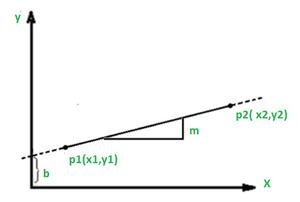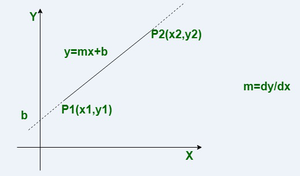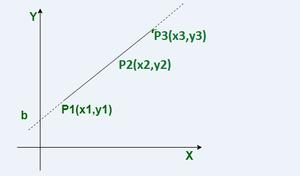# Scan conversion of Line and Line Drawing algorithms

• Last Updated : 08 Jun, 2021

SCAN Conversion of Line :

• A line connects two points.
• It is a basic element in graphics.
• You’ll need two spots between which to draw a line to draw a line .

A line, or line segment, can be uniquely described by two points, according to geometry. We also know from algebra that a line can be defined by a slope, commonly denoted by the letter m, and a y-axis intercept, denoted by the letter b. A line in computer graphics is usually defined by two endpoints. However, most line-drawing algorithms calculate the slope and y- intercept as intermediate outputs.

Line Drawing algorithms :
Given the inherent restrictions of a raster display, the purpose of every line drawing method is to produce the best feasible  approximation of an ideal line. Before getting into specific line drawing algorithms, it’s a good idea to think about the needs  for such algorithms in general.

The primary design criteria are as follows.

• Straight lines appear as straight lines.
• Straight lines start and end accurately.
• Displayed lines should have constant brightness along their length, independent of the line length and orientation.
• Lines should be drawn rapidly.

Method-1 : Direct Method :
In this algorithm, we have two endpoints.  We find the slope of the line by using both the points, and we put the slope in the line equation y = mx + b.

Then we find the value of b by putting x and y equal to 0. After this, we have a relation between x and y. Now we increase the value of x and find the corresponding value of y.
These values will be the intermediate points of the line. After finding the intermediate points we’ll plot those points and draw the line.FIG – Direct method

Method-2 : DDA (Digital Differential Analyzer) Algorithm :
The incremental technique is used in this algorithm. It means that we can find the next coordinates by using past coordinates as a guide. In this method, the difference of pixel point is analyzed and according to the analysis, the line can be drawn.

We’ll start with the initial position and work our way to the ending position by looking for intermediate places. The slope of the line will be the ratio of difference of y-coordinates and the difference of x-coordinates.

```Δy = ( y2 -y1 ), Δx = (x2 - x1)
where, (x1, y1) and (x2, y2) are the endpoints.```

The Digital Differential Analyzer algorithm is based on the values of Δx and Δy.

`Δy = m * Δx , Δx = Δy / m`

The value of the slope will be either positive or negative. If the value of the slope is positive then the values of Δx and Δy are increased otherwise their values are decreased.

```         (i).   If (m < 1): xN = x1 + 1 ,  yN = y1 + m
(ii).  If (m > 1): xN = x1 + 1 / m ,  yN = y1 +1
(iii).  If (m = 1): xN = x1 + 1 , yN = y1 + 1 ```FIG  = DDA algo

Method-3 : Bresenham’s Line Generation :
Another incremental scan conversion procedure is the Bresenham’s algorithm. The big advantage of this algorithm is that, it uses only integer calculations.

This method’s calculation is incredibly quick, which is why the line is drawn swiftly. We’ll need the two endpoints in this and then we have to find the decision parameters.

Let assume that (x1,y1) and (x2,y2) are the two points.

`So, dx = x2-x1 and dy = y2-y1`

The formula for the decision parameter is: di = 2dy – dx.

```           ->   If di >= 0:
Plotted points are,
di +1 = di + 2dy - 2dx  ( i+1 is in base of d )
xN = x1 + 1  ,  yN = y1 + 1
-> If di < 0:
Plotted points are,
di +1 = di + 2dy  ( i+1 is in base of d )
xN = x1 + 1  , yN = y1 ```Fig = Bresenham’s line algorithm

My Personal Notes arrow_drop_up